Journal DetailsFormat
Journal
eISSN
2083-134X
First Published
16 Apr 2011
Publication timeframe
4 times per year
Languages
EnglishOpen Access

# Effect of changing P/Ge and Mn/Fe ratios on the magnetocaloric effect and structural transition in the (Mn,Fe)2 (P,Ge) intermetallic compounds

###### Accepted: 24 May 2016
Journal DetailsFormat
Journal
eISSN
2083-134X
First Published
16 Apr 2011
Publication timeframe
4 times per year
Languages
English

The magnetocaloric effect in the MnxFe2−xP1−yGey intermetallic compounds with the amount of Mn in the range of x = 1.05 to 1.17 and amount of Ge in the range of y = 0.19 to 0.22 has been studied. It was found that a higher Ge/P ratio causes an increase in Curie temperature, magnetocaloric effect at low field (up to 1 T), activation energy of structural transition and a decrease in thermal hysteresis, as well as transition enthalpy. Contrary to this observation, higher Mn/Fe ratio causes a decrease in Curie temperature, slight decrease of magnetocaloric effect at low magnetic field, and an increase in thermal hysteresis. Simultaneous increase of both ratios may be very advantageous, as the thermal hysteresis can be lowered and magnetocaloric effect can be enhanced without changing the Curie temperature. Some hints about optimization of the composition for applications at low magnetic fields (0.5 T to 2 T) have been presented.

#### Keywords

Introduction

Manganese based intermetallic compounds with Fe2P type crystalline structure can be useful for energy applications. These rare-earth-element-free magnetocaloric materials are good candidates for the next generation of regenerators in magnetic cooling devices. Magnetocaloric effect (MCE) can be defined as an effect of temperature change in adiabatically isolated material when exposed to a changing magnetic field. Adiabatic temperature change can be described by the relation: $ΔTad=−∫BiBfTCB∂M∂TBdB$$$\Delta {{T}_{ad}}=-\int\limits_{{{B}_{i}}}^{{{B}_{f}}}{\frac{T}{{{C}_{B}}}{{\left( \frac{\partial M}{\partial T} \right)}_{B}}{dB}}$$ where T denotes Curie point, CB is a heat capacity in the vicinity of magnetic transition at the magnetic field with an induction B, and the integral $ΔSM=∫BiBf(∂M∂T)BdB$$\Delta {{\rm{S}}_{\rm{M}}} = \int\limits_{{B_i}}^{{B_f}} {{{\left( {{{\partial {\rm{M}}} \over {\partial T}}} \right)}_B}dB}$ is the magnetic entropy change. The magnetic entropy change ΔSM is a good parameter to compare usefulness of magnetocaloric compounds, however more appropriate is a comparison of ΔTad which depends on SM, specific heat and temperature of magnetic transition.

Interesting properties of MnFe(P1−xYx) compounds were for the first time reported by Tegus et al.  in MnFeP0.45As0.55. In their other paper, the authors have shown an impact of changing P/As ratio on the Curie temperature and magnetic entropy change . In further studies arsenic was substituted for germanium, which caused a drop in the MCE and an increase in Tc . In all the studied compounds first order magnetic transition (FOMT) has been reported. Until now, several papers describing magnetocaloric effect in the MnFePGe series with respect to the composition changes have been published .

The main goal of the present work is to study the character of structural changes during the phase transition. The impact of the Ge/P and the Mn/Fe ratio on the kinetics of transformation, thermal hysteresis and MCE has been examined.

Experimental

Approximately 5 g samples were prepared by mixing in a mortar high purity Mn 3N, Fe 3N, Mn3P2 3N, Fe2P 3N and Ge 5N, 325 mesh elements and compounds. The powders were pressed at the ambient temperature and a pressure of about 1 GPa. The pressed pellets were sintered for 5 hours at 1273 K and homogenized for the next 50 hours at 923 K under the vacuum of 5 × 10−3 Pa (wire vacuum furnace, Czylok, Poland). Thereafter the samples were slowly cooled down in the furnace to the room temperature. The phase purity and the crystal structures were determined by powder X-ray diffraction (XRD) using AgK radiation (D/MAX RAPID II-R Rigaku Denki Co. Ltd., Japan). The microstructures were observed by energy dispersive spectroscopy (EDS) and wavelength dispersive spectroscopy (WDS) (JCXA 733, JEOL, Japan). Magnetic measurements were performed using a Physical Property Measurement System (PPMS-7, Quantum Design, Inc., USA). The magnetic entropy changes ΔS(T,B) were calculated from isothermal magnetization curves (MB curves) in the vicinity of Curie temperature using the thermodynamic Maxwell relation. The isothermal magnetization curves were measured in a temperature range of 150 K to 350 K under magnetic fields of 0 T to 7 T. The direct adiabatic temperature changes ΔTad(T,B) were obtained with an adiabatic magnetocalorimeter (AMT&C Group, Russia) in a temperature range of 170 K to 350 K under magnetic fields up to 1.7 T. The external magnetic field was changed in a cycle 0 T – 1.7 T – 0 T with a rate of 1 T/s. ΔTad measurements were performed on the samples that were initially milled, mixed with the consolidating wax-based “CEREOX” powder in the ratio 85:15 wt.% and then pressed under 1 GPa pressure. Tablets prepared by this method were resistant to the large magneto-volume effect during the measurements but exhibited lower maximum ΔTad than the bulk samples. Calorimetric studies were performed with the use of Netzsch Pegasus 404c thermal analyzer. Samples with a mass of approximately 30 mg were heated at the rates 5 K/min to 30 K/min under the helium atmosphere with gas flow of 50 mL/min.

Results and discussion

Energy dispersive spectroscopy (EDS) is very effective in showing the presence of microsegregation regions. Additionally, the EDS maps for all the samples (Fig. 1 shows the EDS map of a selected sample) have given the phase composition in the microscale and the elemental analysis for each phase. For all the samples the dominating phase is of Fe2P-type (>80 %), while there are about 5 % of FeMnGe and MnO phases as impurities. Based on such analysis and the chemical composition of the magnetocaloric Fe2P-type phase, the samples have been listed as follows: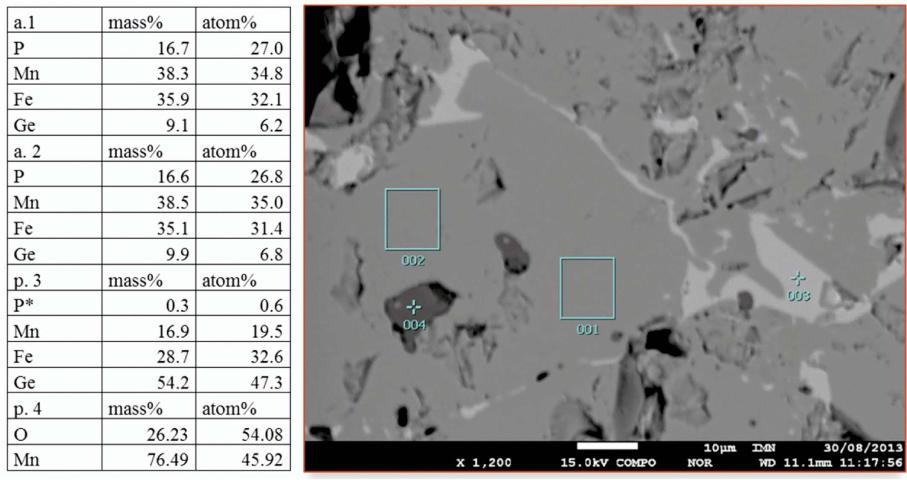Microstructure of sample 1 and its local elemental analysis. In the description a. 1 and a. 2 stand for areas and p. 3 and p. 4 for points.

1. Mn1.05Fe0.95P0.81Ge0.19

2. Mn1.05Fe0.95P0.79Ge0.21

3. Mn1.05Fe0.95P0.78Ge0.22

4. Mn1.1Fe0.9P0.78Ge0.22

5. Mn1.17Fe0.83P0.78Ge0.22

The room temperature powder X-ray diffraction patterns of the studied compounds are shown in Fig. 2. They can be indexed in the hexagonal Fe2P-type structure with the P –6 2 m space group. There are also indications of two impurity phases: ferromagnetic Fe3Mn4Ge6-type (the P m m m space group) and MnO (the F m –3 m space group). The weight fractions of the phases, lattice parameters, unit-cell volume and c/a ratio for Fe2P-type phase have been refined using Rietveld method and presented in Table 1. The selected Rietveld refinement pattern for sample 1 is shown in Fig. 3. Four compositions 1, 2, 4, 5 are paramagnetic at room temperature, whereas sample 3 is ferromagnetic at room temperature. Its smaller c lattice parameter in comparison with sample 1 and 2, and its smaller c/a ratio results from the magnetostriction effect occurring at the Curie temperature, what stays in agreement with the previous results [4, 5].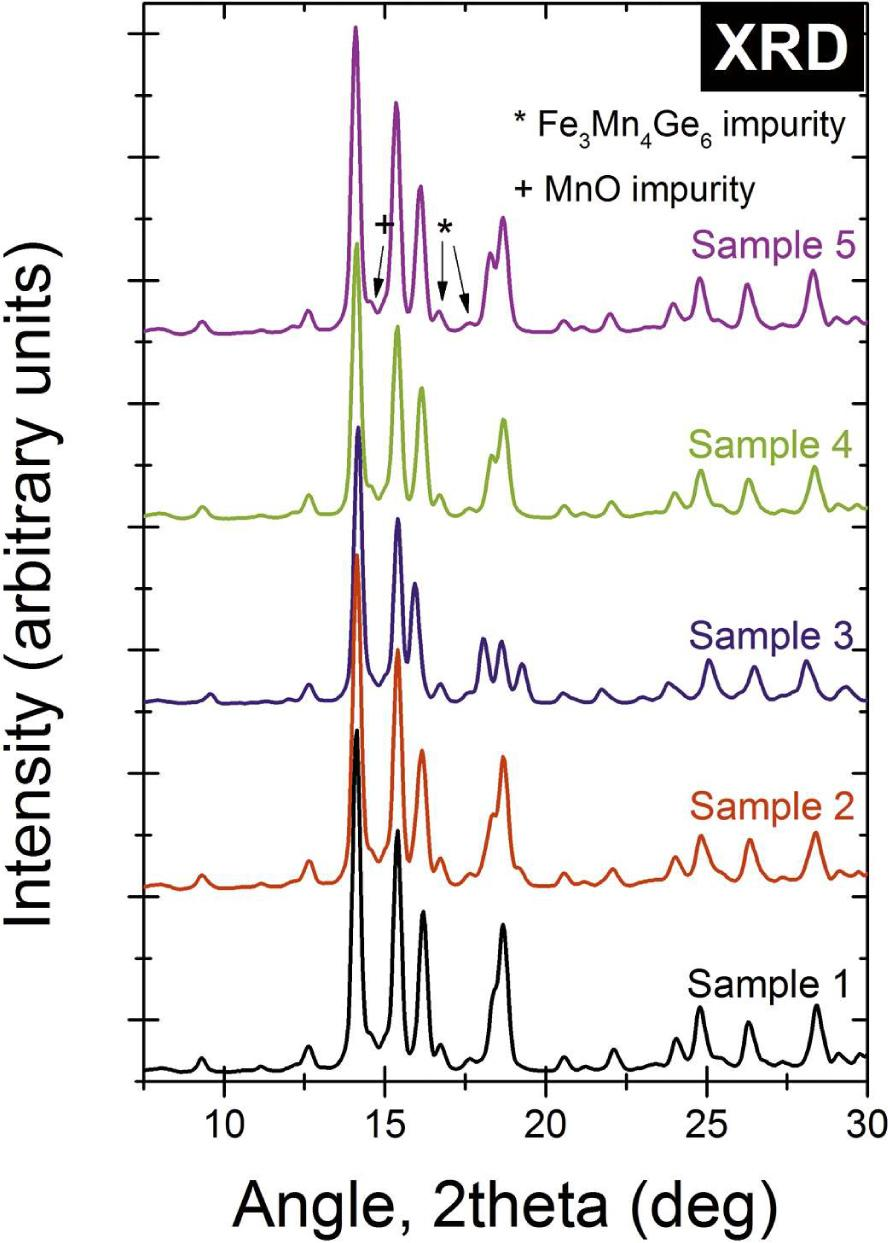Ambient temperature X-ray diffraction patterns.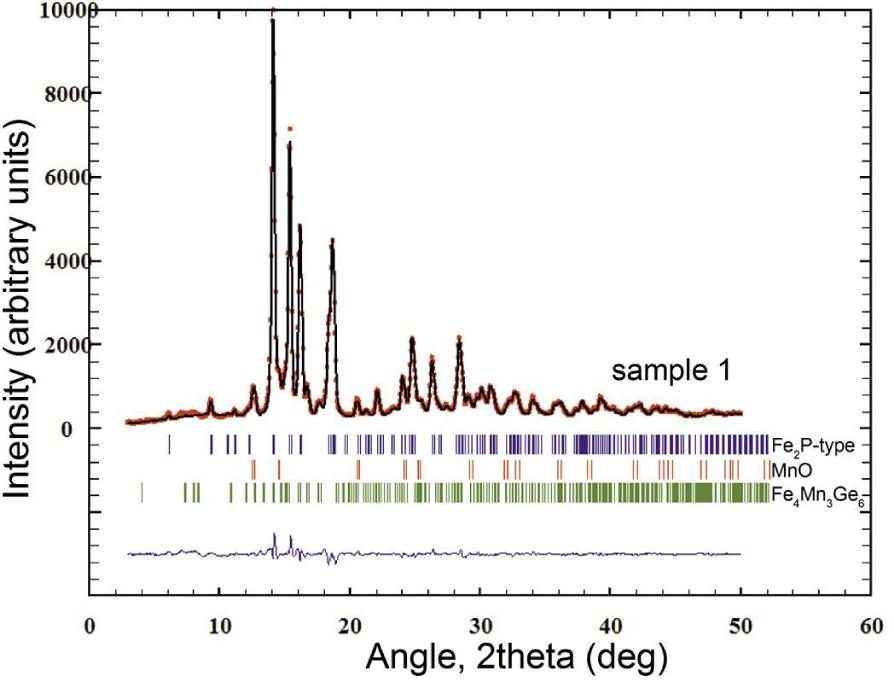Rietveld refinement pattern for Sample 1. Red points – experimental data, solid black line – refined curve, vertical lines define the Bragg line positions separately for each phase, upper blue line marks the difference between the experimental and refined data.

Weight fractions of refined phases, room temperature lattice parameters, c/a ratio and unit-cell volume for Fe2P-type phase. Sample 3 is in the low-temperature ferromagnetic state.

No.Fe2P-type [%]MnO [%]Fe3Mn4Ge6[%]a = b [Å]c [Å]c/a ratioV[ Å3]
187.97±0.746.45±0.535.57±0.176.0795±0.00063.4515±0.00040.5677110.476±0.021
287.53±1.036.57±1.145.90±0.326.1016±0.00143.4421±0.00100.5641110.979±0.049
383.05±1.0712.20±0.974.75±0.216.1765±0.00113.3514±0.00060.5426110.724±0.034
480.97±1.0412.45±0.986.58±0.226.1038±0.00093.4441±0.00060.5704111.123±0.030
581.83±1.3914.24±0.933.93±0.186.1118±0.00083.4429±0.00050.5633111.375±0.027

The temperature dependent powder X-ray diffraction patterns in 2 theta range from 12° to 20° of the samples 1, 3 and 5 are shown in Fig. 4. It is clearly seen that the peak positions are changing continuously during the heating, which indicates that the phase transition is of magnetoelastic type.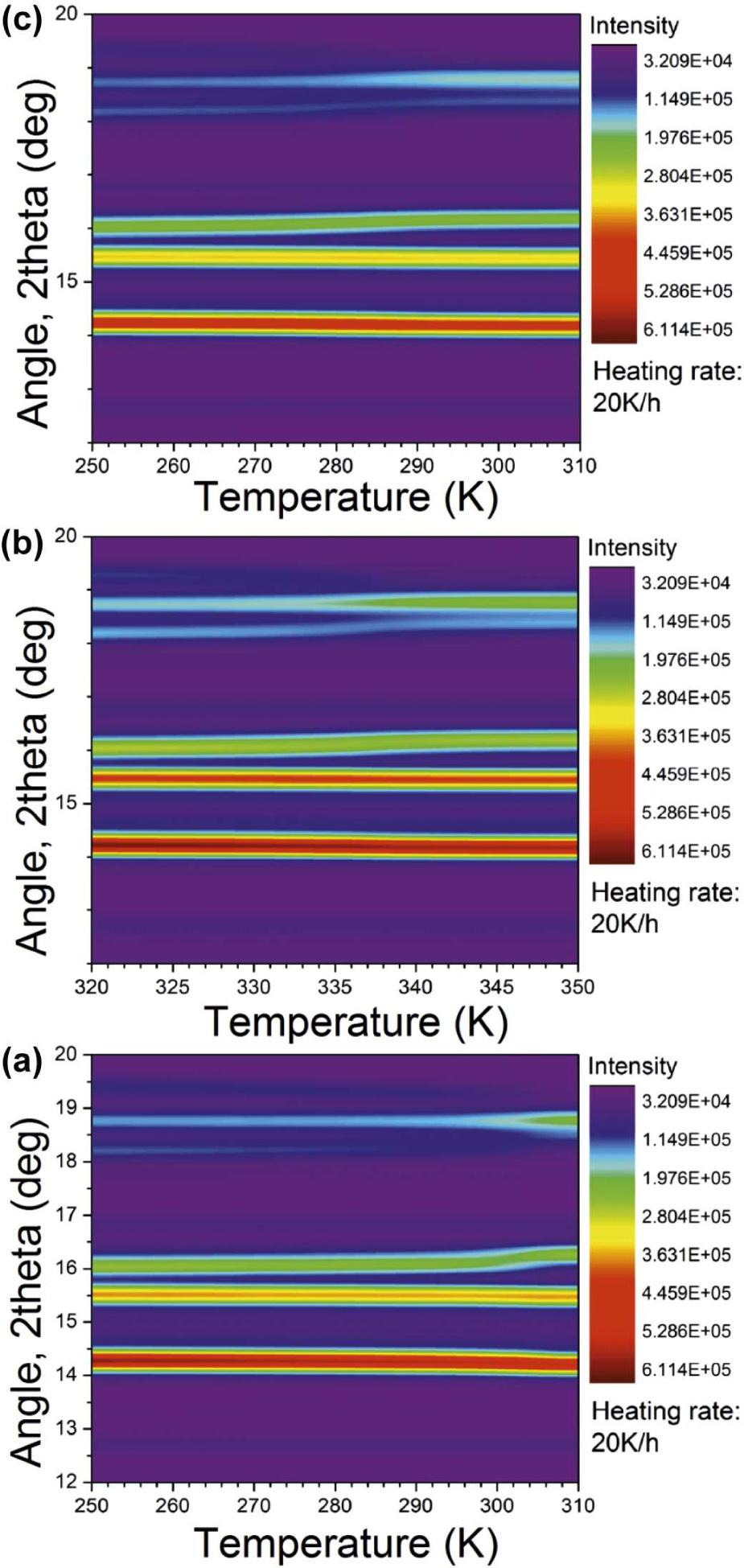Temperature dependent X-ray diffraction maps: (a) Sample 1 (b) Sample 3 and (c) Sample 5.

Magnetic measurements in the magnetic field up to 7 T were performed on three of the five studied samples, i.e. Mn1.05Fe0.95P0.81Ge 0.19 (1), Mn1.05Fe0.95P0.78Ge0.22 (3), and Mn1.17Fe0.83P0.78Ge0.22 (5). Based on these results, effects of changing Mn/Fe as well as P/Ge ratios can be evaluated. In Fig. 5 one can see Arrott plots and magnetic entropy change plots for all three samples. The Arrott plots were prepared with the use of critical exponents adapted from the mean field theory i.e. β = 0.5 and γ = 1. In all three cases the curves on the plots have a negative slope, therefore according to the Banerjee criterion  all these magnetic phase transitions can be classified as a first order type (FOMT). On the first site, the highest negative slope and therefore the lowest value of derivative can be observed in the sample Mn1.05Fe0.95P0.81Ge0.19. Therefore, it can be stated that with a decrease in the Ge/P ratio the first order character of magnetic transition is enhanced while the change in the Mn/Fe ratio barely influences the character of the transition. Along with the Arrott plots, very interesting could be a comparison of magnetic entropy change curves. In the high magnetic field of 5 T, the highest entropy change was evaluated for the Mn1.05Fe0.95P0.81Ge0.19 i.e. 19 J/(kg·K). In the Mn1.05Fe0.95P0.78Ge0.22 the magnetic entropy was found to be 16 J/(kg·K) at B = 5 T, while in the Mn1.17Fe0.83P0.78GeF0.22 it was even lower i.e. 14 J/(kg·K) at the same magnetic field. The opposite situation was observed in low magnetic fields 1 T to 2 T. In the magnetic field equal to B = 2 T, the highest magnetic entropy change (8.5 J/(kg·K)) was observed for the sample with higher amount of germanium i.e. in Mn1.05Fe0.95P0.78Ge0.22. In the compound with an overall formula Mn1.05Fe0.95P0.81Ge0.19 the magnetic entropy change is lower and it is equal to 7.8 J/(kg·K). The magnetic entropy change in magnetic fields in the range of 1 T to 7 T can be observed in Fig. 5. For samples 1, 3 and 5, Curie temperatures are presented in the insets of the Arrott plots in the figure.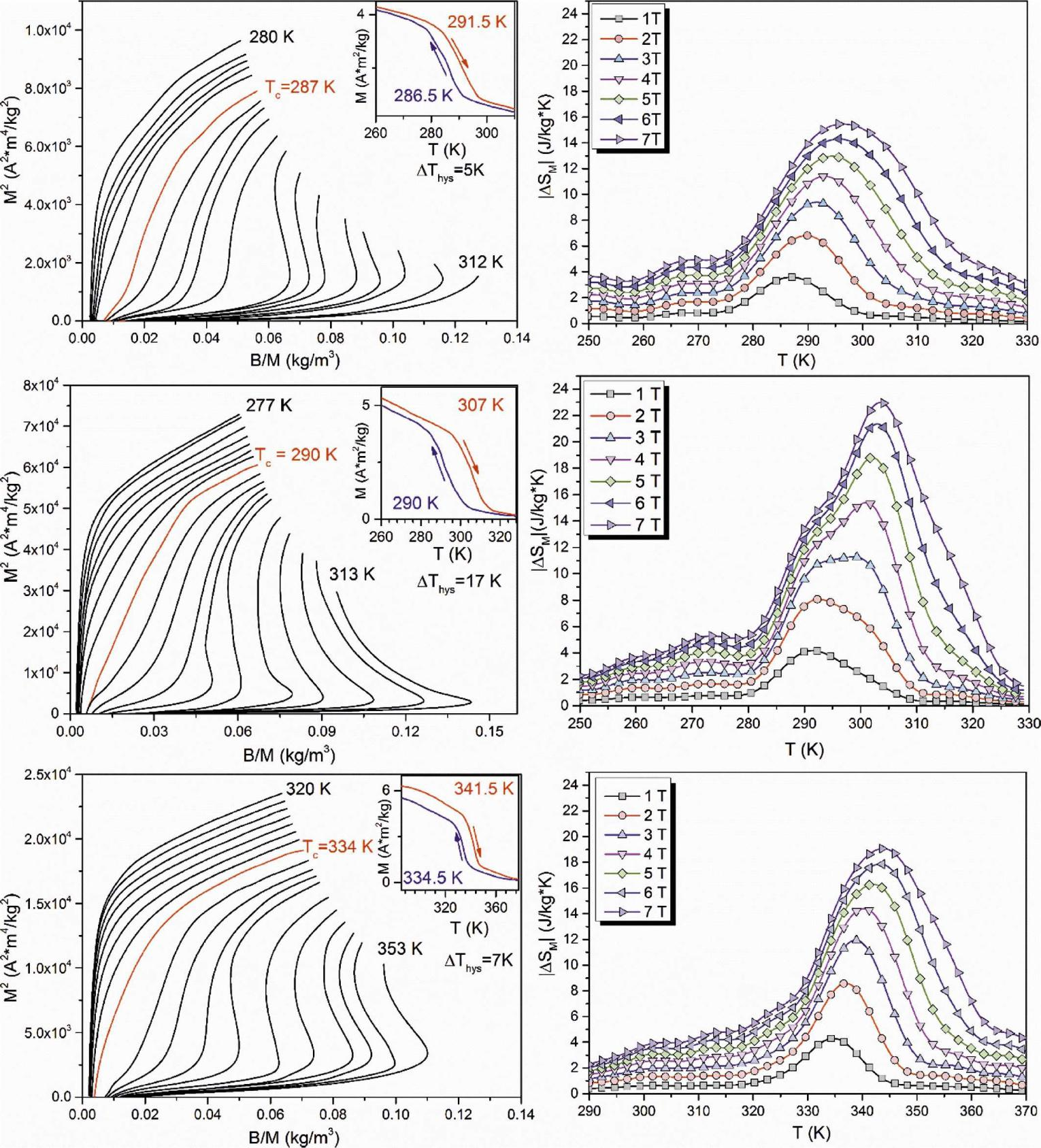Arrott plots and magnetic entropy change for Samples 5, 1 and 3 (numbered from the top)

The second important parameter that describes magnetocaloric effect is adiabatic temperature change. Until now, there have been few studies on adiabatic temperature change in MnFePGe compounds, including direct ΔTad measurements [7, 8]. In the literature , the authors have presented ΔTad measurements of a bulk sample with a composition Mn1.2Fe0.8P0.75Ge0.25. They have obtained maximal ΔTad equal to 1.8 K in magnetic field of 1.1 T. Contrary to the magnetic entropy change, adiabatic temperature change is directly related to the efficiency of the active magnetic refrigerator that could be used in the cooling device. Adiabatic temperature change of the tablets pressed under 1 GPa pressure with 15 wt.% of wax based powder was measured in the field changing up to 1.7 T. Due to the low density and high porosity of prepared samples, magnetocaloric effect may be significantly lower than in the high density bulk alloys of the same type. Despite this fact, the dependence of ΔTad on the composition has been satisfactorily examined in this paper. In Fig. 6, ΔTad(T) curves are presented for all the studied compounds. In the upper panel, the influence of Ge/P ratio, while in the bottom panel, the influence of Mn/Fe ratio on the magnetocaloric effect under the low magnetic field (B = 1.7 T) are presented. As one can see, an increase of germanium amount in the compound (from 0.19 to 0.22) improved the maximum value of ΔTad (from 0.65 K to 1.2 K) despite weakening the first order transition character. Moreover, the weakened first order transition had an advantageous effect of lowering thermal hysteresis of transition. On the other hand, increasing the Mn/Fe ratio caused a slight drop in the amplitude of ΔTad(T) (from 1.2 to 1.0 K) and an increase of thermal hysteresis. It might be interesting to compare Curie temperatures obtained from magnetic measurements and transition temperatures observed from direct ΔTad measurements. There is about 2 K difference between exact Curie temperatures and temperatures at which ΔTad(T) has the maximum value. Moreover, thermal hysteresis values obtained from direct ΔTad measurements are slightly (3 K) higher than those obtained from Curie point measurements. It can be caused by the different heating/cooling rates.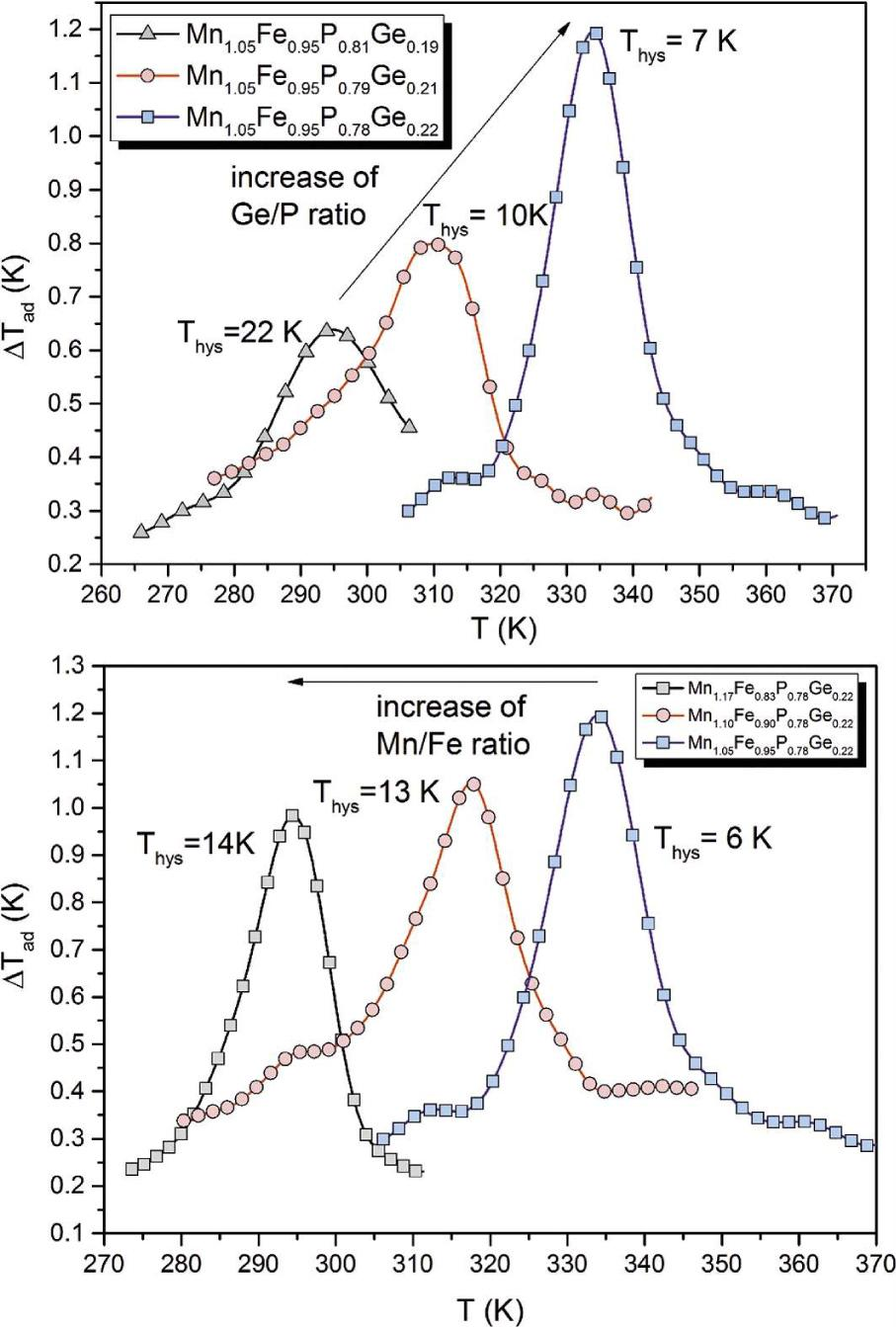Impact of Ge/P and Mn/Fe ratios on adiabatic temperature change and thermal hysteresis of studied samples.

As there is a large difference in magnetocaloric properties between samples with different amount of germanium, calorimetric studies on the structural transition in samples 1 and 3 i.e. Mn1.05Fe0.95P0.78Ge0.22 and Mn1.05Fe0.95P0.78Ge0.19 were carried out. Kinetic parameters, such as rate constants and activation energies were derived from calorimetric measurements by performing heating at different rates (5 K/min to 30 K/min). In order to analyze this solid-solid transition, the models widely used for crystallization such as Avrami and Flyn-Wall-Ozawa were used.

Rate constants were obtained with the use of Avrami model. According to this model under isothermal conditions, degree of reaction (transformation) is given by the equation : $α(t)=1−e−ktn$$$\alpha (t)=1-{{e}^{-k{{t}^{n}}}}$$ where k is a rate constant and n is Avrami exponent. These two parameters can be obtained from the intercept and slope of the double logarithmic form of mentioned equation: $In[−In(1-α(t))]=In(k)+n⋅In(t)$$$\text{In}\left[ -\text{In(1-}\alpha (t)) \right]=\text{In(}k\text{)+}n\cdot \text{In(}t\text{)}$$

As in our case we have non-isothermal conditions, Jeziorny’s modifications  need to be applied. The rate constant k has to be corrected by the heating rate in the following manner: $In(k′)=In(k)Φ$$$\text{In}\left( k\prime \right)=\frac{\text{In(}k\text{)}}{\Phi }$$ where Φ denotes rate of heating. Therefore, half-time of transformation is given by the equation: $t1/2=(In2k′)1/n$$${{t}_{1/2}}={{\left( \frac{\text{In2}}{k\prime } \right)}^{1/n}}$$

In order to determine activation energy as a function of degree of transformation, Flyn-Wall-Ozawa (FWO) model  was used. It is based on Doyle approximation for heterogeneous reactions: $log(Φ)=log(AEaR)−log[g(α(t)]−2.315−0.4567EaRT$$$\begin{array}{*{35}{l}} \log (\Phi ) & = & \log \left( \frac{A{{E}_{a}}}{R} \right)-\log \left[ g(\alpha (t) \right] \\ {} & {} & -2.315-0.4567\frac{{{E}_{a}}}{RT} \\ \end{array}$$

Activation energy can be obtained from the slope of log(Φ) versus 1/T curve. Degree of transformation (α(t)) needed for both models was calculated from DSC measurements by the use of the procedure: $α(t)=∫T0T(dHdT)dT∫T0Tf(dHdT)dT$$$\alpha (t)=\frac{\int\limits_{{{T}_{0}}}^{T}{\left( \frac{dH}{dT} \right)dT}}{\int\limits_{{{T}_{0}}}^{{{T}_{f}}}{\left( \frac{dH}{dT} \right)dT}}$$

In the presented equation, dH/dT is the enthalpy at an infinitesimal temperature, T0 is the onset temperature, while Tf is the final temperature of transformation. Consequently, total time of transformation is given by the relation: $t=T−T0Φ$$$t=\frac{T-{{T}_{0}}}{\Phi }$$

In Table 2, Avrami parameters calculated for two samples with different Ge/P ratios are shown.

Peak temperatures and Avrami kinetic parameters derived for two measured samples with different heating rates. F – rate of heating, Tp – peak transformation temperature, ln(k’) – rate constant logarithm, n – Avrami exponent, t1/2 – half-time of transformation.

Φ [K/min]Tp [K]ln(k’)nt1/2 [min]
Mn1.05Fe0.95P0.78Ge 0.22
30342.48(8.59±0.27) × 10−24.31±0.110.900
25342.337.38±0.28) × 10−24.26±0.120.902
20341.85(5.60±0.30) × 10−24.07±0.120.901
15341.71(−4.31±2.47) × 1034.24±0.130.918
10341.57(−2.11±0.07) × 1014.38±0.160.965
5340.79− 1.01±0.044.46±0.191.155
Mn1.05Fe0.95P0.81Ge 0.19
30305.27(3.37±0.09) × 10−25.24±0.080.926
25304.98(2.10±0.50) × 10−25.18±0.120.928
20304.64(−5.37±0.10) ×1025.27±0.110.942
15304.30(−1.41±0.03) × 1014.87±0.110.955
10303.90(−4.71±0.10) × 1015.25±0.121.020
5303.28−1.66±0.04−5.27±0.131.278

As one can see, there is a slight difference in the value of speed of transformation which is indicated by the half time of process. Structural transformation is slower in the sample with higher amount of Ge. Moreover, there is a difference in the Avrami exponent, which is related to the mechanism of structural change. In our case it could be caused by the more pronounced change of lattice parameters during transition in the sample with germanium amount xGe = 0.19. In Fig. 7, activation energies for transitions in both studied samples obtained from the FWO model have been presented. Activation energy for the same transformation progress (α = 50 %) is equal to 340 and 230 J/(kg·K) for Mn1.05Fe0.95P0.78Ge0.22 and Mn1.05Fe0.95P0.81Ge0.19, respectively. However, an average enthalpy calculated from DSC curves for the same samples is equal to 4.4 J/g and 5.5 J/g, respectively. Therefore, the sharper the first order transition, the lower the activation energy, higher enthalpy and higher Avrami exponent is observed. Additionally to kinetics studies, specific heat near the Curie point for samples 1, 3 and 5 was obtained. It is equal to about 0.63 J/(kg·K) at 300 K, which is 3 times higher than the specific heat of pure gadolinium (0.21 J/(kg·K)).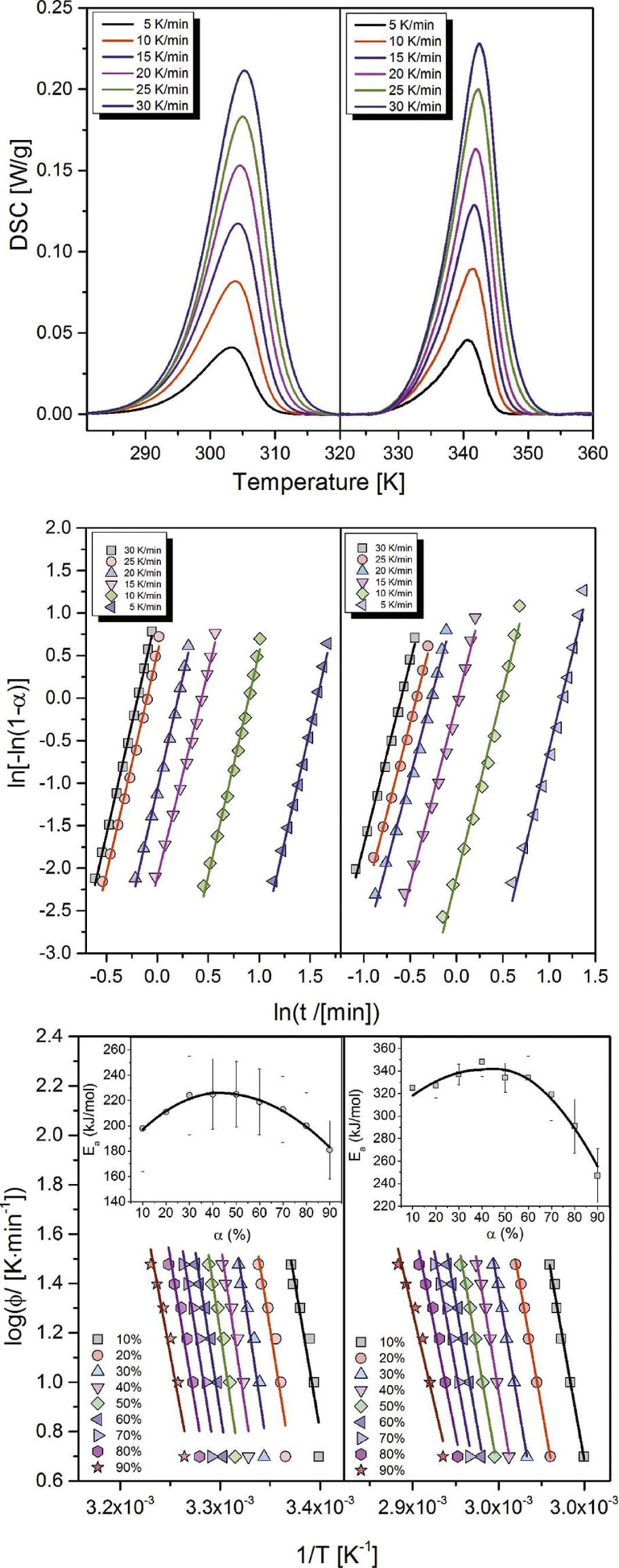Complex analysis of structural transition kinetics in Samples 1 and 3 with different Ge/P ratios. Top panel – DSC curves for different heating rates, middle panel – Avrami fits of kinetic curves, bottom panel – FWO fits and activation energies.

Very interesting is the impact of composition on the thermal hysteresis. As was stated in the previous section, activation energy and enthalpy are related to the strength of first order transition. An increase of activation energy connected with a decrease of enthalpy of the process is related to the suppression of the first order type transition. It was found that the higher Ge/P ratio influences the transition character, which changes gradually into second order type. Thermal hysteresis rises significantly, while lowering the Ge/P ratio (from 7 K to 22 K) which is caused by the stronger first order transition. Surprisingly, an increase of Mn/Fe ratio causes an increase of thermal hysteresis (from 6 K to 14 K), however, the effect is lower than in case of Ge/P ratio change.

In the literature, Mn1.1Fe0.9P0.78Ge0.22 is presented as the best possible composition optimized for magnetic cooling devices at room temperature (Table 3). It has maximum of magnetic entropy change in the magnetic field of 5 T at room temperature. However, the composition should be optimized for lower magnetic fields (0.5 T to 1 T). Our studies indicate that an increase of germanium amount causes significant reduction of thermal hysteresis and increase of magnetocaloric effect in low magnetic field (up to 1 T). The increase of germanium content causes also significant increase of Curie temperature. On the other hand, this temperature can be shifted back to room temperature by increasing the manganese amount.

Comparison of the obtained data with the literature.

Chemical compositionTC [K]DS : BPreparation methodYear
Mn1.1Fe0.9P0.81Ge0.1926014 J/kg K : 2 Tbulk (mechanical alloying)2008
Mn1.1Fe0.9P0.78Ge0.2229820 J/kg K : 2 T
Mn1.1Fe0.9P0.75Ge0.2533013 J/kg K : 2 T
Mn1.1Fe0.9P0.8Ge0.220615 J/kg K : 2 Tribbon bulk alloy (induction) ribbon2006
Mn1.1Fe0.9P0.76Ge0.2429911 J/kg K : 2 T
Mn1.1Fe0.9P0.76Ge0.2431717 J/kg K : 2 T
Mn1.1Fe0.9P0.8Ge0.225528 J/kg K : 2 Tbulk (mechanical alloying)2009
Mn1.1Fe0.9P0.8Ge0.225022 J/kg K : 2 Tbulk (mechanical alloying)2010
MnFeP0.7Ge0.3360bulk (mechanical alloying)2004
MnFeP0.5Ge0.5570
Mn1.1Fe0.9P0.74Ge0.2632345 J/kg K : 5 Tribbons (arc melting + melt spinning)2015
Mn1.1Fe0.9P0.7Ge0.336019 J/kg K : 5 T
Mn1.1Fe0.9P0.68Ge0.324039 J/kg K : 5 T
Mn1.1Fe0.9P0.7Ge0.3380bulk (mechanical alloying)2005
Mn1.15Fe0.85P0.76Ge0.2526310 J/kg K : 2 Tbulk (arc melting)2015
Mn1.15Fe0.85P0.74Ge0.26272
Mn1.15Fe0.85P0.72Ge0.28314
Mn1.15Fe0.85P0.70Ge0.3033012 J/kg K : 2 T
Mn1.15Fe0.85P0.68Ge0.3234212 J/kg K : 2 T
Mn1.05Fe0.95P0.81Ge0.192959 J/kg K : 2 Tbulk (solid-state reaction)this work
Mn1.05Fe0.95P0.79Ge0.21310
Mn1.05Fe0.95P0.78Ge0.223357 J/kg K : 2 T
Mn1.1Fe0.9P0.78Ge0.22318
Mn1.17Fe0.83P0.78Ge0.222939 J/kg K : 2 T
Summary

Impact of Ge/P and Mn/Fe ratios in the MnFePGe intermetallic compounds on the phase transition and magnetocaloric effect has been studied. Despite the fact that decreasing Ge/P ratio causes strengthening of the first order transition, it has adverse effect on the magnetocaloric characteristics in the low magnetic field. Compounds with higher amount of germanium have not only higher magnetocaloric effect in the field up to 2 T but also much lower thermal hysteresis of transition and much higher Curie point. In order to bring Curie point back to room temperature, Mn/Fe ratio can be increased. Therefore, optimal MnFePGe compound for the application in magnetic refrigeration technology at room temperature should be composed of high amounts of both germanium [y(Ge) > 0.22] and manganese [x(Mn) > 1.17].

#### Comparison of the obtained data with the literature.

Chemical compositionTC [K]DS : BPreparation methodYear
Mn1.1Fe0.9P0.81Ge0.1926014 J/kg K : 2 Tbulk (mechanical alloying)2008
Mn1.1Fe0.9P0.78Ge0.2229820 J/kg K : 2 T
Mn1.1Fe0.9P0.75Ge0.2533013 J/kg K : 2 T
Mn1.1Fe0.9P0.8Ge0.220615 J/kg K : 2 Tribbon bulk alloy (induction) ribbon2006
Mn1.1Fe0.9P0.76Ge0.2429911 J/kg K : 2 T
Mn1.1Fe0.9P0.76Ge0.2431717 J/kg K : 2 T
Mn1.1Fe0.9P0.8Ge0.225528 J/kg K : 2 Tbulk (mechanical alloying)2009
Mn1.1Fe0.9P0.8Ge0.225022 J/kg K : 2 Tbulk (mechanical alloying)2010
MnFeP0.7Ge0.3360bulk (mechanical alloying)2004
MnFeP0.5Ge0.5570
Mn1.1Fe0.9P0.74Ge0.2632345 J/kg K : 5 Tribbons (arc melting + melt spinning)2015
Mn1.1Fe0.9P0.7Ge0.336019 J/kg K : 5 T
Mn1.1Fe0.9P0.68Ge0.324039 J/kg K : 5 T
Mn1.1Fe0.9P0.7Ge0.3380bulk (mechanical alloying)2005
Mn1.15Fe0.85P0.76Ge0.2526310 J/kg K : 2 Tbulk (arc melting)2015
Mn1.15Fe0.85P0.74Ge0.26272
Mn1.15Fe0.85P0.72Ge0.28314
Mn1.15Fe0.85P0.70Ge0.3033012 J/kg K : 2 T
Mn1.15Fe0.85P0.68Ge0.3234212 J/kg K : 2 T
Mn1.05Fe0.95P0.81Ge0.192959 J/kg K : 2 Tbulk (solid-state reaction)this work
Mn1.05Fe0.95P0.79Ge0.21310
Mn1.05Fe0.95P0.78Ge0.223357 J/kg K : 2 T
Mn1.1Fe0.9P0.78Ge0.22318
Mn1.17Fe0.83P0.78Ge0.222939 J/kg K : 2 T

#### Peak temperatures and Avrami kinetic parameters derived for two measured samples with different heating rates. F – rate of heating, Tp – peak transformation temperature, ln(k’) – rate constant logarithm, n – Avrami exponent, t1/2 – half-time of transformation.

Φ [K/min]Tp [K]ln(k’)nt1/2 [min]
Mn1.05Fe0.95P0.78Ge 0.22
30342.48(8.59±0.27) × 10−24.31±0.110.900
25342.337.38±0.28) × 10−24.26±0.120.902
20341.85(5.60±0.30) × 10−24.07±0.120.901
15341.71(−4.31±2.47) × 1034.24±0.130.918
10341.57(−2.11±0.07) × 1014.38±0.160.965
5340.79− 1.01±0.044.46±0.191.155
Mn1.05Fe0.95P0.81Ge 0.19
30305.27(3.37±0.09) × 10−25.24±0.080.926
25304.98(2.10±0.50) × 10−25.18±0.120.928
20304.64(−5.37±0.10) ×1025.27±0.110.942
15304.30(−1.41±0.03) × 1014.87±0.110.955
10303.90(−4.71±0.10) × 1015.25±0.121.020
5303.28−1.66±0.04−5.27±0.131.278

#### Weight fractions of refined phases, room temperature lattice parameters, c/a ratio and unit-cell volume for Fe2P-type phase. Sample 3 is in the low-temperature ferromagnetic state.

No.Fe2P-type [%]MnO [%]Fe3Mn4Ge6[%]a = b [Å]c [Å]c/a ratioV[ Å3]
187.97±0.746.45±0.535.57±0.176.0795±0.00063.4515±0.00040.5677110.476±0.021
287.53±1.036.57±1.145.90±0.326.1016±0.00143.4421±0.00100.5641110.979±0.049
383.05±1.0712.20±0.974.75±0.216.1765±0.00113.3514±0.00060.5426110.724±0.034
480.97±1.0412.45±0.986.58±0.226.1038±0.00093.4441±0.00060.5704111.123±0.030
581.83±1.3914.24±0.933.93±0.186.1118±0.00083.4429±0.00050.5633111.375±0.027

Tegus O., Bruck E., Buschow K.H.J., Boer DE F.R.,Nature, 415 (2002), 150.TegusO.BruckE.BuschowK.H.J.Boer DEF.R.Nature415200215010.1038/415150aSearch in Google Scholar

Tegus O., Bruck E., Zhang L., Dagula W., Buschow K.H.J., De Boer F.R.,Physica B, 319 (2002), 174.TegusO.BruckE.ZhangL.DagulaW.BuschowK.H.J.De BoerF.R.Physica B319200217410.1016/S0921-4526(02)01119-5Search in Google Scholar

Li W.X., Tegus O., Zhang L., Dagula W., Bruck E., Buschow K.H.J., Boer De F.R.,IEEET. Magn., 39 (2003), 3148.LiW.X.TegusO.ZhangL.DagulaW.BruckE.BuschowK.H.J.Boer DeF.R.IEEET. Magn392003314810.1109/TMAG.2003.816039Search in Google Scholar

Sougrati M.T., Hermann R.P., Grandjean F., Long G.J., Brück E., Tegus O., Trung N.T., Buschow K.H.J.,J. Phys.-Condens. Mat., 20 (2008), 475206.SougratiM.T.HermannR.P.GrandjeanF.LongG.J.BrückE.TegusO.TrungN.T.BuschowK.H.J.J. Phys.-Condens. Mat20200847520610.1088/0953-8984/20/47/475206Search in Google Scholar

Yabuta H., Umeo K., Takabatake T., Koyama K., Watanabe K.,J. Phys. Soc. Jpn., 75 (2006), 113707.YabutaH.UmeoK.TakabatakeT.KoyamaK.WatanabeK.J. Phys. Soc. Jpn75200611370710.1143/JPSJ.75.113707Search in Google Scholar

Banerjee S. K.,Phys. Lett., 12 (1964), 16.BanerjeeS. K.Phys. Lett1219641610.1016/0031-9163(64)91158-8Search in Google Scholar

Yibole H., Guillou F., Zhang L., Van Dijk N.H., Bruck E.,J. Phys. D Appl. Phys., 47 (2014), 075002.YiboleH.GuillouF.ZhangL.Van DijkN.H.BruckE.J. Phys. D Appl. Phys47201407500210.1088/0022-3727/47/7/075002Search in Google Scholar

Trung N.T., Klaasse J.C.P., Tegus O., Cam Thanh D.T., Buschow K.H.J., Bruck E.,J. Phys. D Appl. Phys., 43 (2010), 015002.TrungN.T.KlaasseJ.C.P.TegusO.Cam ThanhD.T.BuschowK.H.J.BruckE.J. Phys. D Appl. Phys43201001500210.1088/0022-3727/43/1/015002Search in Google Scholar

Avrami M.,J. Chem. Phys., 7 (1939), 1103.AvramiM.J. Chem. Phys71939110310.1063/1.1750380Search in Google Scholar

Jeziorny A.,Polymer, 19 (1978), 1142.JeziornyA.Polymer191978114210.1016/0032-3861(78)90060-5Search in Google Scholar

Flynn J.H., Wall L.A.,J. Polym. Sci. Lett., 4 (1966), 323.FlynnJ.H.WallL.A.J. Polym. Sci. Lett4196632310.1002/pol.1966.110040504Search in Google Scholar

Ozawa T.,B. Chem. Soc. Jpn., 38 (1965), 1881.OzawaT.B. Chem. Soc. Jpn381965188110.1246/bcsj.38.1881Search in Google Scholar

Doyle C.D.,Nature, 207 (1965), 290.DoyleC.D.Nature207196529010.1038/207290a0Search in Google Scholar

• #### Growth, spectral, optical, dielectric and third order nonlinear studies on 2-amino 4-methylpyridinium salicylate single crystals

Recommended articles from Trend MD